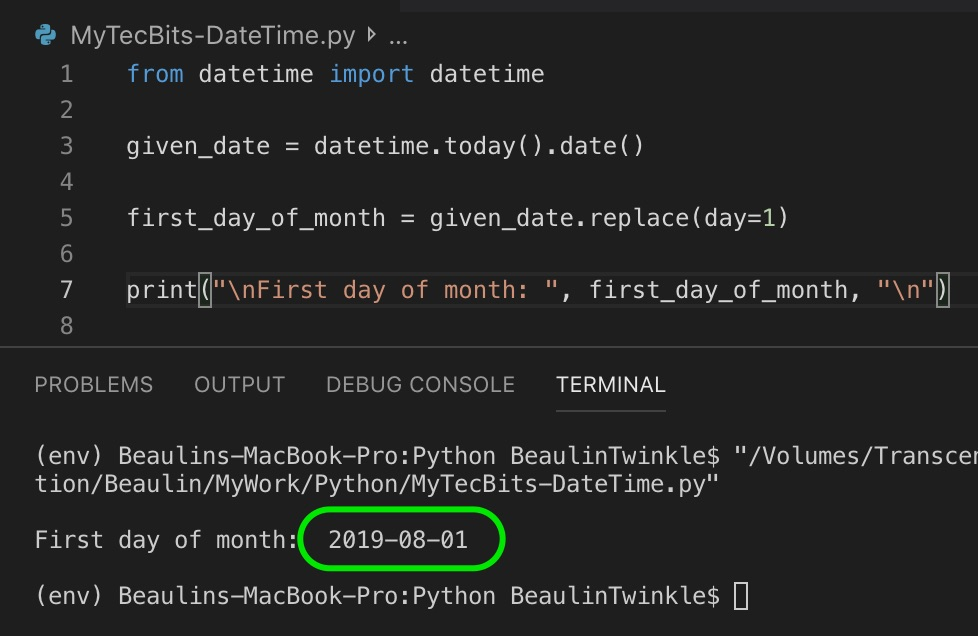# How to find first day of the month in Python?

In today’s article of python tip series, we will see a quick code sample on how to find the first day of the month from the given date. There is no straight forward way to get month’s first day in Python. We have to apply some simple logic to get it. Here are few of the methods.

## Method 1:

In this, the date.replace() method of the datetime module is used to replace the date part of the date object to 1.

```from datetime import datetime

given_date = datetime.today().date()

first_day_of_month = given_date.replace(day=1)

print("\nFirst day of month: ", first_day_of_month, "\n")
```

## Method 2:

In this, the timedetla of the datetime module is used to find the days from the first day to the given date and then subtract it from the given date to get the first day.

```from datetime import datetime, timedelta

given_date = datetime.today().date()

first_day_of_month = given_date - timedelta(days = int(given_date.strftime("%d"))-1)

print("\nFirst day of month: ", first_day_of_month, "\n")
```

## Method 3:

In this method, strftime() is used to format the date object with the day part as 01, which returns the first day of the month.

```import time
from datetime import datetime

given_date = datetime.today().date()

first_day_of_month = given_date.strftime("%Y-%m-01")

print("\nFirst day of month: ", first_day_of_month, "\n")
```

## Reference

### 1 thought on “How to find first day of the month in Python?”

1.get 1s day of every month of given year
import datetime
import calendar
import re
from datetime import date
def findDay(date):
born = datetime.datetime.strptime(date, ‘%Y %m %d’).weekday()
return (calendar.day_name[born])

def hyp(j):

no_hyphens = re.sub(‘-‘,’ ‘,str(j))
return no_hyphens

def send(year_start):
date = hyp(year_start)
print (date)
print(findDay(date))

epoch_year = 2019
for i in range(1,13):
year_start = date(epoch_year, i, 1)
send(year_start)

Reply

This site uses Akismet to reduce spam. Learn how your comment data is processed.